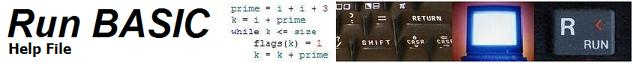IF...THEN...[ELSE]...[END IF]

IF test expression THEN {code goes here}
IF test expression GOTO [branchlabel]

IF test expression THEN {code goes here} ELSE {code goes here}
IF test expression GOTO [branchlabel1] ELSE [branchlabel2]

IF test expression THEN
{code goes here}
END IF

IF test expression THEN
{code goes here}
ELSE
{code goes here}
END IF

Description:

The IF...THEN statement provides a way to change program flow based on a test expression. For example, the following line directs program execution to branch label [soundAlarm] if fuel runs low.

if fuel < 5 then [soundAlarm]

Since the expression after THEN is a branch label, this command may also be written using the GOTO statement instead of the THEN statement.

if fuel < 5 goto [soundAlarm]

Any time the statement after THEN is a branch label, you may use GOTO instead of THEN.

Another way to control program flow is to use the IF...THEN...ELSE statement. This extended form of IF...THEN adds expressiveness and simplifies coding of some logical decision-making software. Here is an example of its usefulness.

Consider:

[retry]
input "Please choose mode, (N)ovice or e(X)pert?"; mode\$
if len(mode\$) = 0 then print "Invalid entry! Retry" : goto [retry]
mode\$ = left\$(mode\$, 1)
if instr("NnXx", mode\$) = 0 then print "Invalid entry! Retry" : goto [retry]
if instr("Nn", mode\$) > 0 then print "Novice mode" : goto [main]
print "eXpert mode"
[main]

The conditional lines can be shortened to one line as follows:

if instr("Nn",mode\$)> 0 then print "Novice mode" else print "eXpert mode"

Some permitted forms are as follows:

if a < b then statement else statement
if a < b then [label] else statement
if a < b then statement else [label]
if a < b then statement : statement else statement
if a < b then statement else statement : statement
if a < b then statement : goto [label] else statement
if a < b then gosub [label1] else gosub [label2]

Any number of variations on these formats are permissible. The a < b expression is of course only a simple example chosen for convenience. It must be replaced with the correct expression to suit the problem.

IF...THEN...END IF is another form using what are called conditional blocks. This allows great control over the flow of program decision making. Here is an example of code using blocks.

if qtySubdirs = 0 then
print "None."
goto [noSubs]
end if

A block is merely one or more lines of code that are executed as a result of a conditional test. There is one block in the example above, and it is executed if qtySubdirs = 0.

It is also possible to use the ELSE keyword as well with blocks:

if qtySubdirs = 0 then
print "None."
else
print "Count of subdirectories: "; qtySubdirs
end if

This type of coding is easy to read and understand. There are two blocks in this example. One is executed if qtySubdirs = 0, and one is executed if qtySubdirs is not equal to 0. Only one of the two blocks will be executed (never both as a result of the same test).

These conditional blocks can be nested inside each other:

if verbose = 1 then
if qtySubdirs = 0 then
print "None."
else
print "Count of subdirectories: "; qtySubdirs
end if
end if

In the example above, if the verbose flag is set to 1 (true), then display something, or else skip the display code entirely.